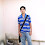# Create Bar Chart in Excel - Java POI Example Program

## Bar Chart - JFreeChart - POI - Introduction

In this tutorial, let us discuss how to create a bar chart in Excel, using a Java Program. We will utilize Apache POI library to read an Excel document that has the chart data. We will use JFreeChart to create the chart and then stamp the chart back to the worksheet using POI. You would need POI / JFreeChart libraries to work with the example in this section.The example provided in this section works for XLS spreadsheets. We will write a separate post to cover XLSX.

## Bar Chart - Data Source

The data source for the bar chart is available in an Excel sheet (barchart.xls).A screenshot of the data is provided below:Java - POI - JFreeChart - Bar Chart - Data Source - Input

## Create Bar Chart in Excel - Java Program Example

The complete Java program that creates a bar chart in Excel worksheet using POI and JFreeChart is provided below:
```import java.io.*;
import org.apache.poi.ss.usermodel.*;
import org.apache.poi.hssf.usermodel.*;
import org.apache.poi.hssf.util.*;
import org.apache.poi.util.IOUtils;
import org.apache.poi.hssf.usermodel.HSSFClientAnchor;
import org.jfree.data.category.DefaultCategoryDataset;
import org.jfree.chart.ChartFactory;
import org.jfree.chart.JFreeChart;
import org.jfree.chart.ChartUtilities;
import org.jfree.chart.plot.PlotOrientation;
import java.util.Iterator;
public class CreateBarChartExample {
public static void main(String[] args) throws Exception{
/* Read the bar chart data from the excel file */
FileInputStream chart_file_input = new FileInputStream(new File("barChart.xls"));
/* HSSFWorkbook object reads the full Excel document. We will manipulate this object and
write it back to the disk with the chart */
HSSFWorkbook my_workbook = new HSSFWorkbook(chart_file_input);
/* Read chart data worksheet */
HSSFSheet my_sheet = my_workbook.getSheetAt(0);
/* Create Dataset that will take the chart data */
DefaultCategoryDataset my_bar_chart_dataset = new DefaultCategoryDataset();
/* We have to load bar chart data now */
/* Begin by iterating over the worksheet*/
/* Create an Iterator object */
Iterator<Row> rowIterator = my_sheet.iterator();
/* Loop through worksheet data and populate bar chart dataset */
String chart_label="a";
Number chart_data=0;
while(rowIterator.hasNext()) {
Row row = rowIterator.next();
//Read cells in Rows and get chart data
Iterator<Cell> cellIterator = row.cellIterator();
while(cellIterator.hasNext()) {
Cell cell = cellIterator.next();
switch(cell.getCellType()) {
case Cell.CELL_TYPE_NUMERIC:
chart_data=cell.getNumericCellValue();
break;
case Cell.CELL_TYPE_STRING:
chart_label=cell.getStringCellValue();
break;
}
}
/* Add data to the data set */
/* We don't have grouping in the bar chart, so we put them in fixed group */
}
/* Create a logical chart object with the chart data collected */
JFreeChart BarChartObject=ChartFactory.createBarChart("Subject Vs Marks","Subject","Marks",my_bar_chart_dataset,PlotOrientation.VERTICAL,true,true,false);
/* Dimensions of the bar chart */
int width=640; /* Width of the chart */
int height=480; /* Height of the chart */
/* We don't want to create an intermediate file. So, we create a byte array output stream
and byte array input stream
And we pass the chart data directly to input stream through this */
/* Write chart as PNG to Output Stream */
ByteArrayOutputStream chart_out = new ByteArrayOutputStream();
ChartUtilities.writeChartAsPNG(chart_out,BarChartObject,width,height);
/* We can now read the byte data from output stream and stamp the chart to Excel worksheet */
/* we close the output stream as we don't need this anymore */
chart_out.close();
/* Create the drawing container */
HSSFPatriarch drawing = my_sheet.createDrawingPatriarch();
/* Create an anchor point */
ClientAnchor my_anchor = new HSSFClientAnchor();
/* Define top left corner, and we can resize picture suitable from there */
my_anchor.setCol1(4);
my_anchor.setRow1(5);
/* Invoke createPicture and pass the anchor point and ID */
HSSFPicture  my_picture = drawing.createPicture(my_anchor, my_picture_id);
/* Call resize method, which resizes the image */
my_picture.resize();
/* Close the FileInputStream */
chart_file_input.close();
/* Write changes to the workbook */
FileOutputStream out = new FileOutputStream(new File("barChart.xls"));
my_workbook.write(out);
out.close();
}
}

```

## Output - Bar Chart Excel - Java Program

The output of the Java Program is provided below. We got a bar chart as expected:Bar Chart in Excel using Java - POI JFreeChart - Output Example

That completes the tutorial to create a bar chart in XLS using POI / JFreeChart. You can try this example and post a comment if you are stuck. We will provide a XLSX version of this tutorial shortly. Stay connected.

1.How to change the displaying subject style in x-axis ?

2.which jars to use

3.Gracias Danke Thank You !!!!!!! :)

4.bookmarked!!, I love your web site!

5.How to make chart for specific data from specific cells in excel